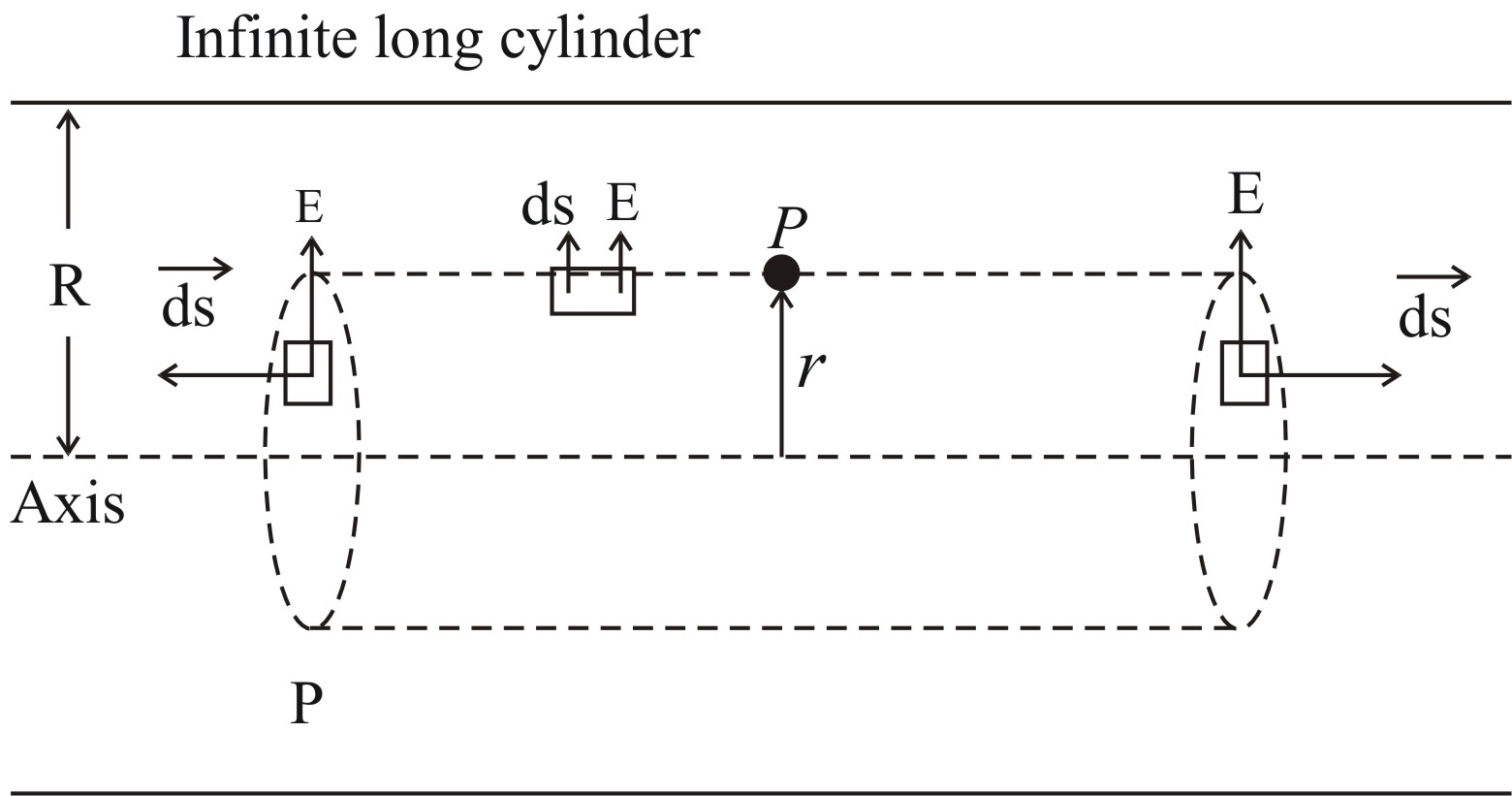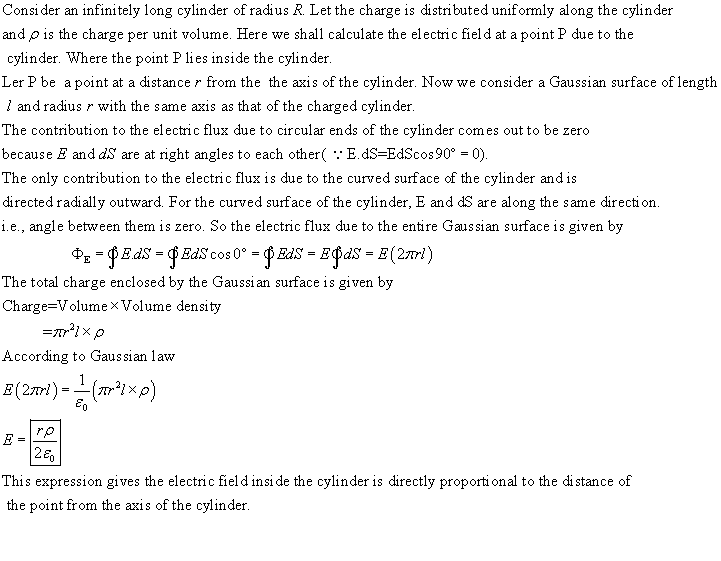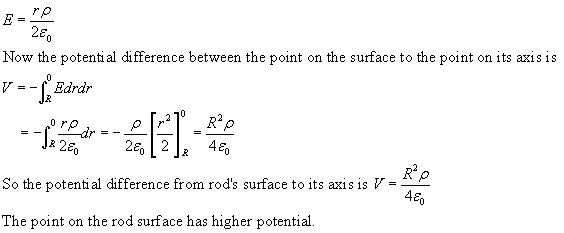Question# An infinitely long rod of radius R carries a uniform volume charge density PKS\rho\ (\rho > 0). a) Show how to use Gauss' Law to prove that the electr

Other
ANSWEREDAn infinitely long rod of radius R carries a uniform volume charge density $$\rho\ (\rho > 0)$$.
a) Show how to use Gauss' Law to prove that the electric field inside this rod points radially outward and has magnitude.
$$\displaystyle{E}={\frac{{\rho\cdot{r}}}{{{2}\cdot\epsilon_{{0}}}}}$$
b) Integrate the electric field over an appropriate displacement to find the potential difference from the rod's surface to its axis. State explicity which of those two locations is at the higher potential.